Search IntMath
Close

450+ Math Lessons written by Math Professors and Teachers

5 Million+ Students Helped Each Year

1200+ Articles Written by Math Educators and Enthusiasts

Simplifying and Teaching Math for Over 23 Years

# Arc length of a spiral around a paraboloid

By Murray Bourne, 17 Jun 2014

## Background

A reader from Africa, Magnar, recently asked about running a string in a spiral around a parabolic dish, as illustrated in the following image: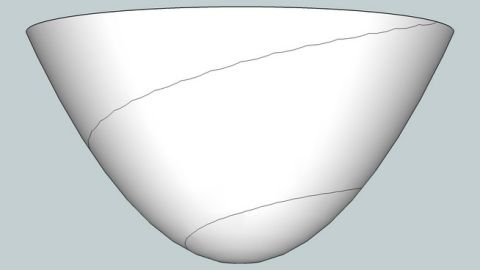Magnar wanted to know how to calculate the length of such a spiral.

There's an interesting story behind Magnar's question. He builds parabolic dish cookers in Africa. In this photo, he's helping to install one of his dishes in a village. The dish concentrates the heat of the sun and the cook pot is placed at the focus of the parabola.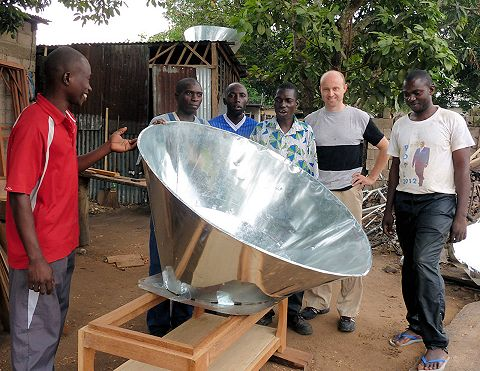(Image used with permission)

This is a great use of sustainable energy, it keeps the air cleaner (by avoiding open fires inside huts), and saves women from the backbreaking and time-consuming task of gathering firewood every day.

Let's look at some of the math behind this problem.

## Paraboloid

A paraboloid is the 3D surface resulting from the rotation of a parabola around an axis. The equation of a simple paraboloid is given by the formula:

z = x2 + y2

The surface generated by that equation looks like this, if we take values of both x and y from −5 to 5: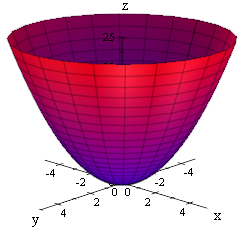Some typical points on this curve are (0,0,0), (1,1,2), (-2,3,13) and (3,4,25). That last point is on the top lip of the surface in the 3-D graph given above, at the front, facing us. (In this graph, the x- and y-axes axes are equally scaled, but the z-axis is not.)

## Using Parametric equations

We can also represent this paraboloid using parametric equations with variables t and r, as follows:

x = r cos(t)

y = r sin(t)

z = r2

We could write this as an ordered triple, like this:

(r cos(t), r sin(t), r2)

We only need to let t take values from 0 to (otherwise we just "paint" the surface more than necessary) and r takes values from 0 to 5 if our aim is to create the same curved surface as shown in the above graph.

## A spiral on the surface

Let's get back to the original problem. We want to create a spiral around the surface of the paraboloid. We modify the earlier parametric equations to get a curve rather than a surface, like this.

x = t cos(t)

y = t sin(t)

z = t2

Once again, we could write this as an ordered triple, as follows:

(t cos(t), t sin(t), t2)

We no longer create a surface with this expression. Instead, it will be a curve.

As the variable t takes various values starting from t = 0, it generates a spiral around the 3-D surface.

Let's see how, starting with a simple case.

## Simple Case - curve in the x-z plane

Let's consider the case where we fix the curve so it is in the x-z plane only (it's not actually spiralling in this case, to make things simple.) We write this as:

(t, 0, t2)

The graph of this is part of a parabola, starting at (0,0,0) and extending to (20,0,400), as shown.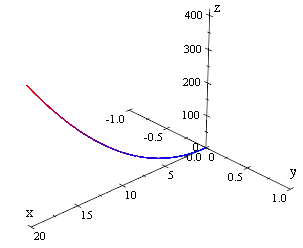What is the length of this simple curve?

## Arc length of a curve

We use a result from calculus, which is based on the Pythagorean Theorem.

The arc (or curved) length is given by: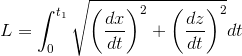(See Arc Length of a Curve and Arc Length of a Curve in Parametric or Polar Coordinates for background on these formulas.)

In this example where x = t and z = t2,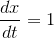and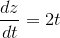Also, in this example we have the end point t1 = 20.

Substituting gives: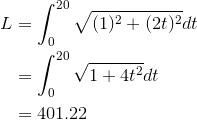(I used a computer algebra system to find this integral.)

This seems quite a low firgure for the length, since the z-value at the end of the curve is 400, and going around the curve should add more than just over 1 unit to the length.

However, the above graph does not have equally-scaled axes, whereas the following one does.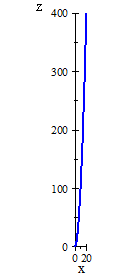We see that a parabola is "almost" straight, and a curve length of just over 400 for this curve is reasonable.

## A simple spiral

Let's now see the effect of those trigonometric terms we saw before.

We now plot (t cos(t), t sin(t), t2) for t = 0 to t = 20. (Of course, t is in radians.)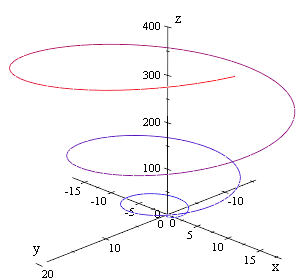The start of the curve, when t = 0, is at the point (0,0,0). The point where the curve finishes, when t = 20, is:

(20 cos(20), 20 sin(20), 202) = (8.162, 18.259, 400).

For interest, looking from above (down along the z-axis), the above spiral looks like this: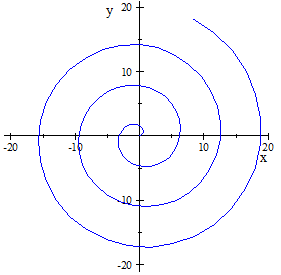The arms cut the x-axis evenly at 2π = 6.28, 4π = 12.57, and 6π = 18.85.

You can see the curve ends near (8, 18) as we claimed before.

## The arc length for a 3-dimensional spiral

We extend the 2-dimensional case above now that we are working in 3 dimensions. In general, the length of an arc when using the parametric terms ((x(t), y(t), z(t)) is given by: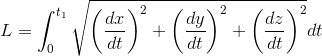Substituting in our expressions for x, y and z, we have: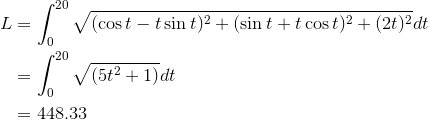## Actual solar cooker paraboloid

Now to examine the solar cooker problem.

Considering one of Magnar's cooker designs, the parabola has a cross-section which is quite flat compared to the paraboloid we drew above. (Like most parabolic dishes, they are using flat panels to approximate the curved surface.)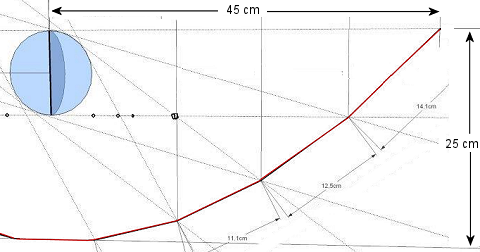In fact, the top lip passes through the point (25,45) in that design. The general form of a parabola can be written:

z = cx2

Substituting (25,45) gives us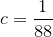.

We now plot the curve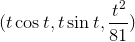from t = 0 to t = 45.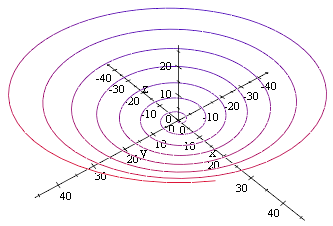The curve starts at (0,0,0) and the top point is (23.6, 38.3, 45). We see there are about 7 spirals.

Here it is from the side: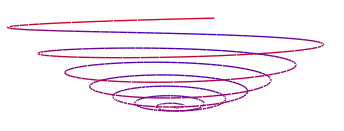And next the view from the top shows we finish near x = 24 and y = 38: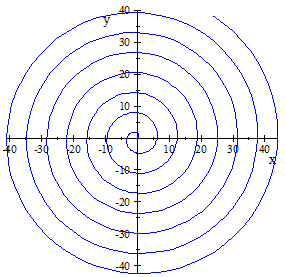## How do we get more or less spirals?

We have around 7 turns in the above spiral, but we may need more or less. If we want to achieve more spirals within the same x-, y-, and z-constraints, we need to multiply the variable within the trigonometric terms by a number.

Here is the case when we multiply the variables in the trig terms by 5. That is: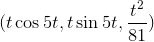We get around 35 spirals this time: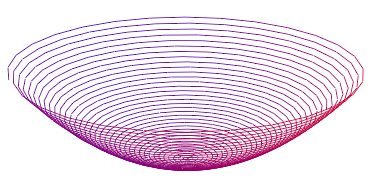Here it is from the side.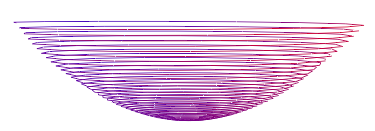The length of this spiral is: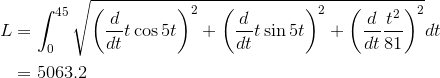Similarly, if we multiply those variables by a number less than 1, we get less spirals. Here is the case when we multiply by 0.3: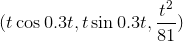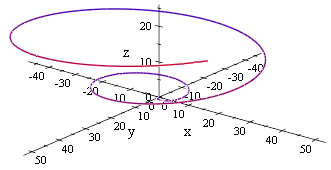This time we have just over 2 spirals (which is similar to the situation we had in Magnar's first diagram at the top) and our length is: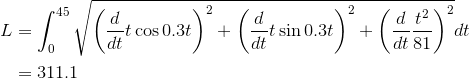## Conclusion

This has been an interesting application of math where 3-D geometry and integral calculus are being used to examine a good use of solar power. We need to make more use of the huge amount of energy available from the sun, and solar cook pots are a great way to do so.

### 12 Comments on “Arc length of a spiral around a paraboloid”

1. R. Jayaram says:

A very useful and interesting article for practical applications. Thank you.

2. Mahesh says:

Isn't there a dt at the end for the curve length integral?

3. Murray says:

@Mahesh: Well spotted! My examples had the "dt" but the 2 general formulas did not.

They do now. Thanks for the feedback.

4. Troy says:

I am looking through your post and a number of the image equations are not showing up in the browser. I am using Firefox 50.1.0.

5. Murray says:

@Troy: Thanks for alerting me! It was a problem on the server end, not with Firefox. I've fixed them now.

6. Troy says:

@Murray, no problem! Excellent article. I have a question, what purpose did Magnar have in wondering what the length of the string traced around the paraboloid would be? Was it simple curiosity or was there a practical purpose? I have read the article a couple of times and it doesn't seem to be mentioned.

The reason I wanted to see the equations is that I am working on a similar problem, the string tracing around a conic spiral. Specifically I wanted to build an LED strip christmas tree controlled by micro controllers and I needed an estimate of the strip length wrapped around the tree (cone). I went off on a tangent and worked out the integral for arc length of the spiral given a particular height and generated a bunch of computer code.

Here is the basic arc length integral: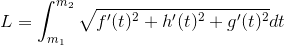Here are the parametric equations: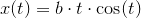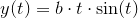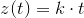Where b is the factor in the distance between successive turns (actually it is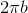)

And their derivatives: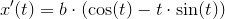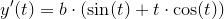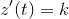The final integral: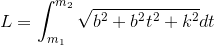What did you use for solving the integrals? I used http://www.integral-calculator.com/ to help me with the derivation and python for the implementation. The solution to the integral is involved and comments are not the correct place for it.

The length that I am getting seems to be reasonable but I would like to confirm that I did it correctly and didn't miss anything. Would you know of a way to approximate the solution as a check for a 3D spiral?

Cheers,
Troy

7. Murray says:

Hi Troy

Magnar was interested in making a spiral solar cooker (see this 3D sketchup).

What you've done looks good to me. To check it, I modeled a conical tree with height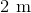and bottom radius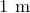. I used the following parametric equation: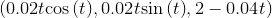With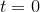to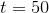, this gives the following conical spiral: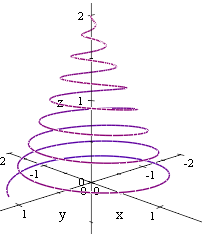Using your formula I got the spiral length to be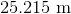, which tallies with the result when using your first (more fundamental) formula.

There are about 8 spirals and looking from above, the gap between each spiral would be about 1/8 = 0.125. This tallies with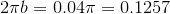from your statement.

So it all seems to make sense.

I used Scientific Notebook (a computer algebra system) to solve it, but you could also do it with Wolfram|Alpha as shown in this solution. (It also provides the indefinite integral answer, if needed.)

Regards
Murray

8. Troy says:

Hi Murray, I appreciate your help!

It is fascinating that Magnar is making use of the spiral line in the fabrication of the cookers. From the 3D model it looks as though the spiral is scribed onto the pieces of sheet metal. Once the sheet metal is cut it can be properly assembled into the solar cooker. What a fantastic practical use of mathematics!

Initially I thought that the spiral problem was a bit of a tangent for me, but like so many things it was quite a pleasant diversion. Thank you for confirming.

I also did somewhat of an approximation that seemed to coincide with the length as well. What I did was to assume that each turn comprised two different approximations of a circle. In the following images you can see the green dots on each turn of the spiral:

Intersection Points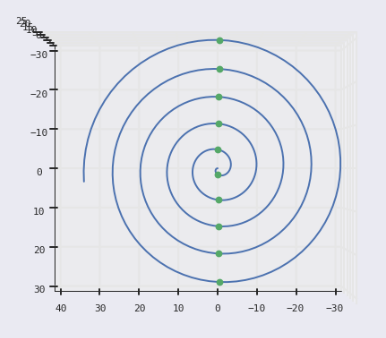Intersection Points - Isometric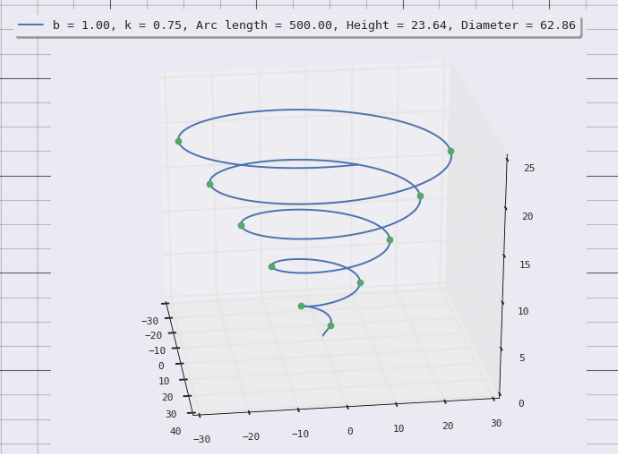I calculated those points as places to have supports for the spiral. Given the x,y & z position:

x = 0.000; y = 1.571; z = 1.178
x = -0.000; y = -4.712; z = 3.534
x = 0.000; y = 7.854; z = 5.890
x = -0.000; y = -10.996; z = 8.247
x = 0.000; y = 14.137; z = 10.603
x = -0.000; y = -17.279; z = 12.959
x = -0.000; y = 20.420; z = 15.315
x = -0.000; y = -23.562; z = 17.671
x = -0.000; y = 26.704; z = 20.028
x = -0.000; y = -29.845; z = 22.384

I can use the absolute value of the y coordinate as a radius of a half circle. We can sum all the half circumferences (pi*r) and arrive at an approximation. When I did it it was pretty close. I suspect that for larger spirals the error will increase.

I haven't heard of Scientific Notebook before, it looks interesting. I have used Wolfram Alpha to help with solving the integral. I tend to use a mix of python and Jupyter Notebooks. Jupyter is very similar to Matlab, except open source.

Cheers,
Troy

9. Anthony says:

Hello,

Could there be a mistake? I calculate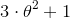instead of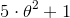in section "The arc length for a 3-dimensional spiral".

Thanks!

Anthony

10. Murray says:

@Anthony. Actually, the variable is, not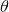.

When expanding the first bracket, we'll get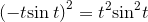From the second bracket we get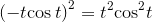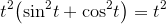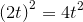and we have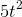.

11. Anthony says:

Sorry for the previous comment. I was the one who made the mistake. Forgot to square the whole derivative.

I am now trying to draw a spiral on a flat sheet of paper to fold/bend it and create a paraboloid approximation.

Here is where I got.
My 2D spiral is defined by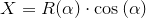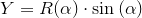For the first turn of the spiral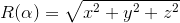with xyz the coordinates of the point on the 3D spiral as you defined it
We get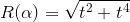Now lets take a xOz plane and start rotating it 20 degrees by 20 degrees and cut the 3D spiral. Every 20 degrees I trace a spoke in the plane between 0 and the intersection of the plane and the spiral.

Now I want to trace those spoke on my flat paper. If I trace them every 20 degrees when I will bend the paper they will not be on my planes.

To know the correspondance between t and alpha that I will use the length of the spirals. For my 3D spiral the length is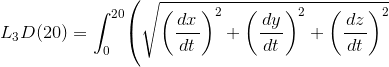And for the 2D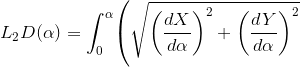We want to know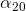so that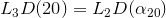I have been lazy here and use wolfram alpha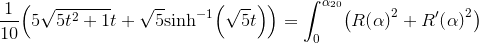with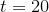degrees

And here I am stuck since I do not have the function R in function of alpha but theta. Do you have any idea on how I could continue?

12. Murray says:

Hi Anthony

I thought about this (and tried a few paper models) and feel that perhaps this problem is in the same category that cartographers face - that is, it's not possible to project such a curved surface on a flat plane. I hope I'm wrong and that you can do this.)

I'm thinking Magnar's approach may be what you should pursue. That is, determine good quadrangular segment approximations (based on circular cross-sections), something like this: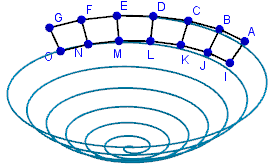I guess another approach is to produce a ribbon (rather than a spiral) whose width would vary. The top of the paraboloid would be quite neat, but the vertex may be a challenge.

### Comment Preview

HTML: You can use simple tags like <b>, <a href="...">, etc.

To enter math, you can can either:

1. Use simple calculator-like input in the following format (surround your math in backticks, or qq on tablet or phone):
a^2 = sqrt(b^2 + c^2)
(See more on ASCIIMath syntax); or
2. Use simple LaTeX in the following format. Surround your math with $$ and $$.
$$\int g dx = \sqrt{\frac{a}{b}}$$
(This is standard simple LaTeX.)

NOTE: You can mix both types of math entry in your comment.